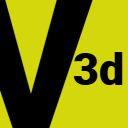# fes2vector3dUser Guide

## Vector 3d utility

`fes2vector3d` is a small library for 3 dimension vectors. A `V3d` is a vector with three `Float` coordenates `x`, `y` and `z`.

### Example

```  fansh> a := V3d(2f, 5f, -4f)
(2.0,5.0,-4.0)

fansh> b := V3d(-2f, -3f, -5f)
(-2.0,-3.0,-5.0)

fansh> c := a + b
(0.0,2.0,-9.0)

fansh> c.magnitude
9.219544457292887

fansh> a * 3
(6.0,15.0,-12.0)

fansh> b * 2.5f
(-5.0,-7.5,-12.5)
```

### Operators

• Addition: `a + b`
• Substraction: `a - b`
• Negation: `-a`
• Scalar multiplication: `a * n`
• Scalar division: `a / n`

### Operations

• Dot product
• Cross product
• Magnitude (or abs or size) ||a||
• Distance